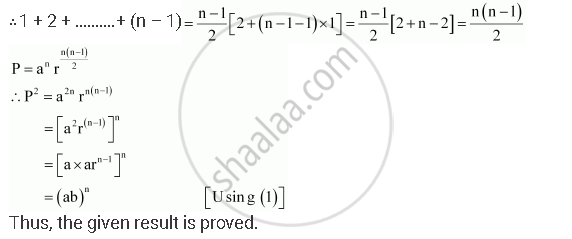CBSE (Arts) Class 11CBSE
Share
Notifications

View all notifications
Books Shortlist
Your shortlist is empty

# If the First and the Nth Term of a G.P. Are a Ad B, Respectively, and If P is the Product of N Terms, Prove that P2 = (Ab)N. - CBSE (Arts) Class 11 - Mathematics

Login
Create free account

Forgot password?
ConceptGeometric Progression (G. P.)

#### Question

If the first and the nth term of a G.P. are a ad b, respectively, and if P is the product of terms, prove that P2 = (ab)n.

#### Solution

The first term of the G.P is a and the last term is b.

Therefore, the G.P. is aarar2ar3, … arn–1, where r is the common ratio.

b = arn–1 … (1)

P = Product of n terms

= (a) (ar) (ar2) … (arn–1)

= (a × a ×…a) (r × r2 × …rn–1)

an r 1 + 2 +…(n–1) … (2)

Here, 1, 2, …(n – 1) is an A.P.Is there an error in this question or solution?

#### APPEARS IN

NCERT Solution for Mathematics Textbook for Class 11 (2018 to Current)
Chapter 9: Sequences and Series
Q: 23 | Page no. 193
Solution If the First and the Nth Term of a G.P. Are a Ad B, Respectively, and If P is the Product of N Terms, Prove that P2 = (Ab)N. Concept: Geometric Progression (G. P.).
S